Affine surfaces parametrized by quadratic polynomials

Introduction
Let p1, p2, p3 be quadratic polynomials in two variables u, v. This means they're of the form
pi(u,v)=au2+buv+cv2+du+ev+f
for constant coefficients a, b, c, d, e, f. If you plot the parametric graph
(x,y,z)=P(u,v)=(p1 , p2 , p3)
for some range of input values u, v, then the image of P is usually a two-dimensional surface in (x,y,z)-space.

If L is a linear transformation of (x,y,z) space, and T is a constant vector, then the transformation A(x,y,z)=L(x,y,z)+T is called an "affine transformation" of space. Similarly, if l is a linear transformation of the (u,v) plane and t is a constant vector in the plane, then a(u,v)=l(u,v)+t is an affine transformation of the plane. It is easy to see that if P(u,v) is a quadratic parametrization as above, then A(P(a(u,v))) is also a quadratic parametrization --- the domain variables are transformed by a, then mapped by P into space, and then transformed by A.

The parametric maps P and Q are "equivalent" means that they are related by invertible affine transformations of domain and target, so Q(u,v)=A(P(a(u,v))). The equivalence classification of parametric maps was found by J. Peters and U. Reif in a 1997 paper. They showed that a map from R2 to R3 must be equivalent to one of fifteen types, or else its image is contained in some plane.

If the image of P is contained in a plane, then, as shown by the paper, there are fifteen types whose image is not contained in a line, five non-constant types whose image is contained in a line, and one type where P is a constant map whose image is a point. There are also five classes of maps from R2 to R4 whose image is not contained in three dimensions, and one map R2 to R5 whose image is not contained in four dimensions, in a class by itself. So, there are a total of 1+5+15+15+5+1=42 types of maps.

For the proof, see the paper of Peters and Reif, The 42 equivalence classes of quadratic surfaces in affine n-space, which appeared in Computer Aided Geometric Design (5) 15 (1998), 459-473.

The fifteen types of non-planar maps R2 to R3 are listed in the illustrated table below, labeled by the Peters-Reif (P-R) code.

Connection to Steiner surfaces
A Steiner surface in R3 is also given by a parametric map involving quadratic polynomials, but there are four: p0, p1, p2, p3, and the polynomial p0 is used as a denominator to get three rational functions: (x,y,z)=(p1/p0 , p2/p0 , p3/p0). The surfaces parametrized by polynomials are simply the special case where p0 is the constant polynomial 1. To see how the affine surfaces fit into the projective classification of Steiner surfaces, one must take into account the "points at infinity" in the domain and target.

 > plot3d([u^2+v^2,u,v],u=-2..2,v=-2..2);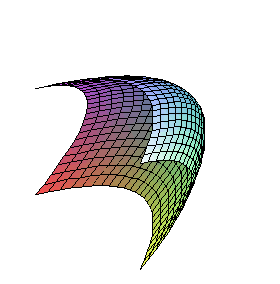P-R Type 3-1-1.

Implicit equation: x-y2-z2=0.

Singularities: none, the image is a smooth graph.

 > plot3d([u*v,u,v],u=-2..2,v=-2..2);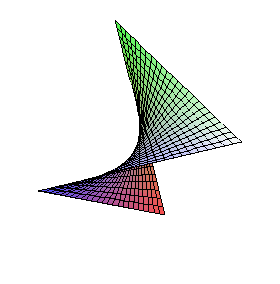P-R Type 3-1-2.

Implicit equation: x-yz=0.

Singularities: none, the image is a smooth graph.

 > plot3d([u^2,u,v],u=-2..2,v=-2..2);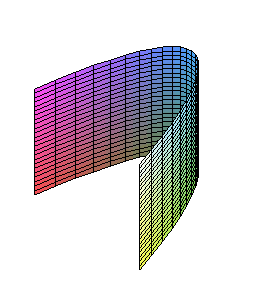P-R Type 3-1-3.

Implicit equation: x-y2=0.

Singularities: none, the image is a smooth graph.

 > plot3d([u^2,v^2,u+v],u=-2..2,v=-2..2);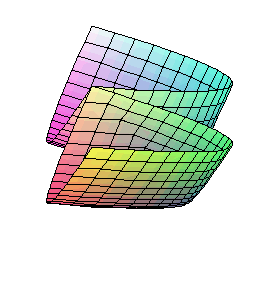P-R Type 3-2-1a.

Steiner surface: Type 5

Implicit equation: x2-2xz2-2xy-2z2y+z4+y2=0.

Singularities: a double line terminating at a pinch point.

The double line of the Type 5 Steiner surface that contains two pinch points and the T-shaped triple point is at infinity.

 > plot3d([u^2,v^2,u],u=-2..2,v=-2..2);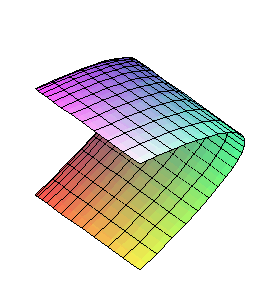P-R Type 3-2-1b.

Implicit equation: x-z2=0.

Singularities: a line of critical points in the domain is mapped to a fold along a parabola in the image.

The parametric image does not cover the whole cylinder, only the intersection of the cylinder with the closed half-space where y is non-negative. Note how the parametric curves are more closely spaced on the edge. The parametric map is two-to-one, except along the singular locus which is mapped to the edge.

 > plot3d([u^2,v^2+u,v],u=-2..2,v=-2..2);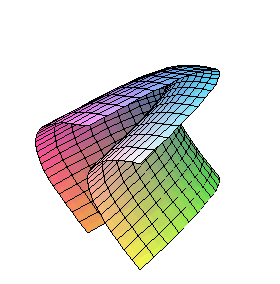P-R Type 3-2-1c.

Steiner surface: Type 6.

Implicit equation: x-y2+2z2y-z4=0.

Singularities: none, the image is a smooth graph.

The "cross cup" surface, with its double line at infinity.

 > plot3d([u^2,u*v,v],u=-2..2,v=-2..2);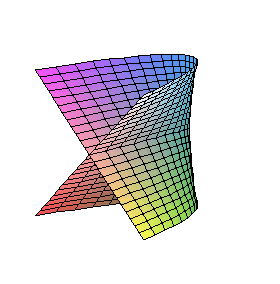P-R Type 3-2-2a.

Cubic Steiner surface: Type 8.

Implicit equation: y2-xz2=0.

Singularities: a double line terminating at a pinch point.

The Whitney Umbrella surface.

More images: 1, 2

 > plot3d([u^2+v,u*v,u],u=-4..4,v=-2..2);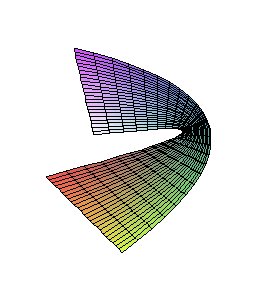P-R Type 3-2-2b.

Cubic Steiner surface: Type 9.

Implicit equation: xz-z3-y=0.

Singularities: none, the image is a smooth graph.

Cayley's ruled cubic, with the double line at infinity.

 > plot3d([u^2,u*v,u],u=-2..2,v=-2..2);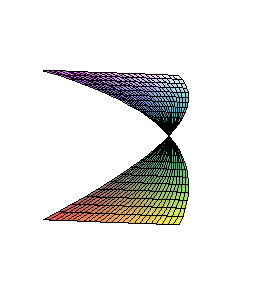P-R Type 3-2-2c.

Implicit equation: x-z2=0.

Singularities: a line of critical points in the domain is mapped to a single point.

The image is the entire cylinder, except the y-axis, one of the generating lines, where only the origin is in the image.

 > plot3d([u^2-v^2,u*v,u],u=-2..2,v=-2..2);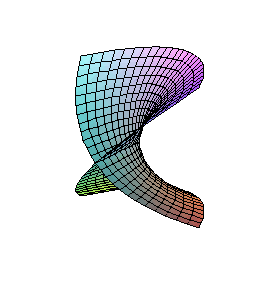P-R Type 3-2-3.

Steiner surface: Type 4.

Implicit equation: y2+xz2-z4=0.

Singularities: a double line terminating at a pinch point.

The double line of the Type 4 surface that contains the T-shaped triple point is at infinity.

 > plot3d([u^2,v^2,u*v],u=-2..2,v=-2..2);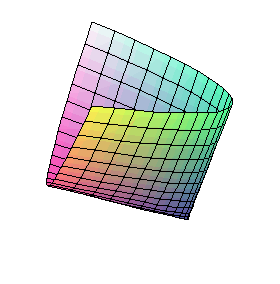P-R Type 3-3-1a.

Implicit equation: xy-z2=0.

Singularities: one critical point in the domain is mapped to the cone's vertex. The parametric map is otherwise two-to-one: P(u,v)=P(-u,-v).

The parametric image is half the quadric cone, including the vertex.

 > plot3d([u^2,v^2,u*v+u],u=-2..2,v=-2..2);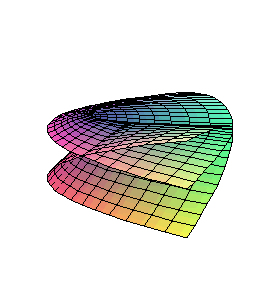P-R Type 3-3-1b.

Steiner surface: Type 5.

Implicit equation: -2xz2+x2y2-2x2y-2xz2y+x2+z4=0.

Singularities: two double lines, each terminating in a pinch point, but along one line, the self-intersection of the image is not transverse and this line also contains the pinch point of the other line - an unstable configuration.

The other two pinch points of the Type 5 Steiner surface are at infinity.

 > plot3d([u^2,v^2,u*v+u+v],u=-2..2,v=-2..2);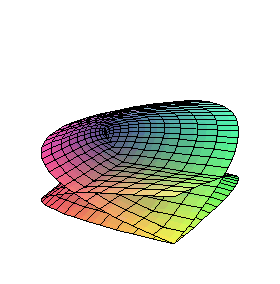P-R Type 3-3-1c.

Steiner surface: Type 1.

Implicit equation: x2y2-2x2y+y2-2xz2y-8zxy+x2-2xz2-2xy+z4-2z2y-2xy2=0.

Singularities: three double lines meeting at a triple point, each ending in a pinch point.

Steiner's Roman surface, with the other three pinch points at infinity.

 > plot3d([u^2,v^2+u,u*v],u=-2..2,v=-2..2);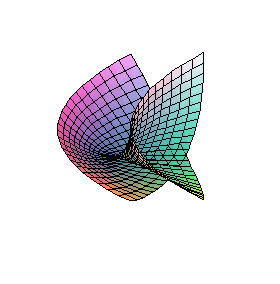P-R Type 3-3-2a.

Steiner surface: Type 6.

Implicit equation: x3-x2y2+2xz2y-z4=0.

Singularities: one double line terminating at a pinch point, but the self-intersection is not transverse.

The "cross cup" surface, with one pinch point at infinity.

 > plot3d([u^2,v^2+u,u*v-v],u=-2..2,v=-2..2);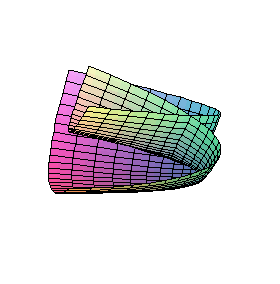P-R Type 3-3-2b.

Steiner surface: Type 3.

Implicit equation: x3-x2y2-2x2+2xy2+2xz2y+4xz2+2z2y-z4+x-y2=0.

Singularities: a double line terminating at a pinch point.

The "cross cap" surface, with one pinch point at infinity.

Some observations
• Steiner surface types 2 and 7 are not represented.
• To identify the affine equivalence class of a non-planar parametric map from R2 to R3, it is enough to look at its image. This is definitely false for maps from R2 to R2, where inequivalent maps can have the same image. Considering the above types 3-1-3, 3-2-1b, and 3-2-2c, it is not enough just to look at some open subset of the image. It is also not enough to look at the implicit equation, which describes the smallest real affine variety containing, but not necessarily equal to, the image.
• Every line in the (u,v) domain is mapped by P to a parabola on the surface. However, some of these parabolas may degenerate into straight lines or rays or points. This means the image surface contains a lot of (possibly degenerate) parabolas - you can see some of them as the grid lines in the Maple plot. A very old-fashioned way to say it is that the image contains ∞2 parabolas. Another description is that at a point in general position on the surface, every line tangent to the surface at that point is also tangent to a parabola contained on the surface.